# Python Debugger – Python pdb

• Difficulty Level : Medium
• Last Updated : 30 Sep, 2022

Debugging in Python is facilitated by pdb module (python debugger) which comes built-in to the Python standard library. It is actually defined as the class Pdb which internally makes use of bdb(basic debugger functions) and cmd (support for line-oriented command interpreters) modules. The major advantage of pdb is it runs purely in the command line, thereby making it great for debugging code on remote servers when we don’t have the privilege of a GUI-based debugger.

pdb supports:

• Setting breakpoints
• Stepping through code
• Source code listing
• Viewing stack traces

## Starting Python Debugger

There are several ways to invoke a debugger

• To start debugging within the program just insert import pdb, pdb.set_trace() commands.  Run your script normally, and execution will stop where we have introduced a breakpoint. So basically we are hard coding a breakpoint on a line below where we call set_trace().  With python 3.7 and later versions, there is a built-in function called breakpoint() which works in the same manner. Refer following example on how to insert set_trace() function.

### Example1: Debugging a Simple Python program of addition of numbers using Python pdb module

Intentional error: As input() returns string, the program cannot use multiplication on strings. Thus, it’ll raise ValueError.

## Python3

 `import` `pdb`  `def` `addition(a, b):``    ``answer ``=` `a ``*` `b``    ``return` `answer`  `pdb.set_trace()``x ``=` `input``(``"Enter first number : "``)``y ``=` `input``(``"Enter second number : "``)``sum` `=` `addition(x, y)``print``(``sum``)`

Output :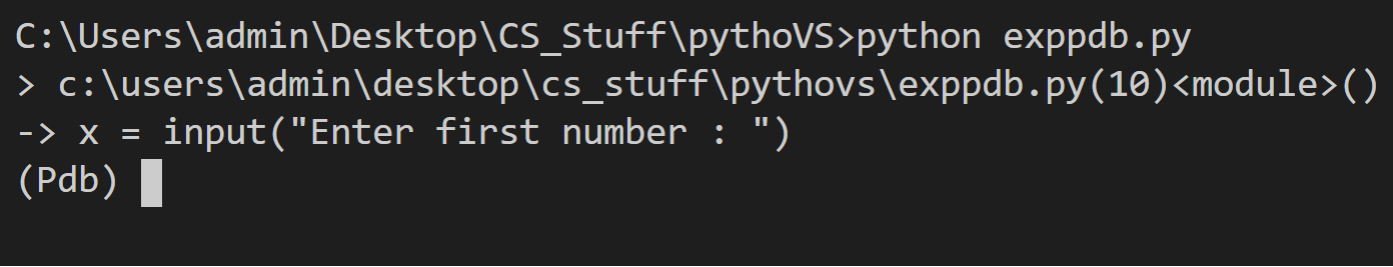set_trace

In the output on the first line after the angle bracket, we have the directory path of our file, line number where our breakpoint is located, and <module>. It’s basically saying that we have a breakpoint in exppdb.py on line number 10 at the module level. If you introduce the breakpoint inside the function, then its name will appear inside <>.  The next line is showing the code line where our execution is stopped. That line is not executed yet. Then we have the pdb prompt. Now to navigate the code, we can use the following commands :

Now, to check the type of variable, just write whatis and variable name. In the example given below, the output of type of x is returned as <class string>. Thus typecasting string to int in our program will resolve the error.

### Example 2: Checking variable type uisng pdb ‘whatis’ command

We can use ‘whatis‘ keyword followed by a variable name (locally or globally defined) to find its type.

## Python3

 `a ``=` `20``b ``=` `10` `s ``=` `0``for` `i ``in` `range``(a):``      ``# this line will raise ZeroDivision error``    ``s ``+``=` `a ``/` `b``    ``b ``-``=` `1`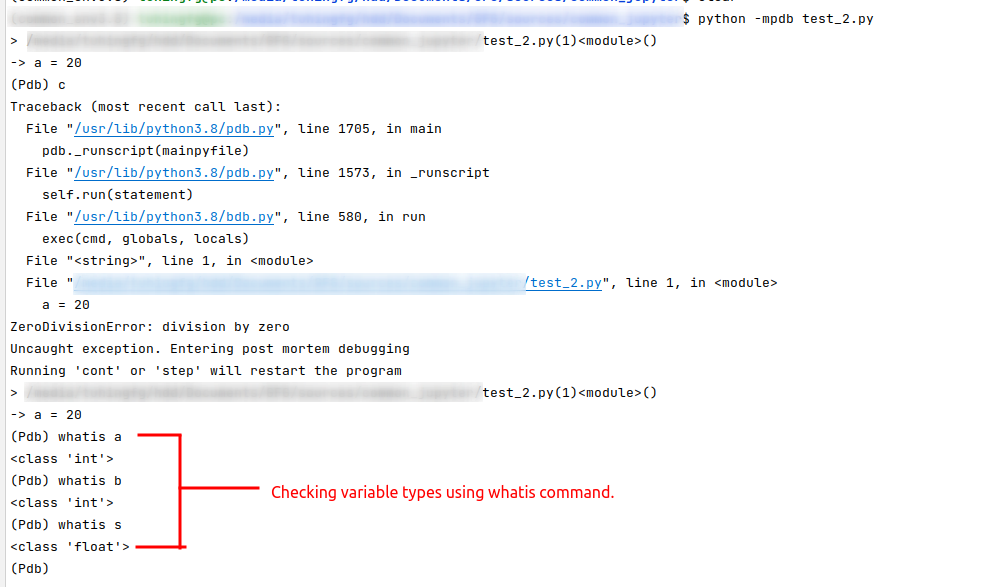Finding variable type using whatis command in pdb

• From the Command Line: It is the easiest way of using a debugger. You just have to run the following command in terminal

python -m pdb exppdb.py (put your file name instead of exppdb.py)

This statement loads your source code and stops execution on the first line of code.

### Example 3: Navigating in pdb prompt

We can navigate in pdb prompt using n (next), u (up), d (down). To debug and navigate all throughout the Python code, we can navigate using the mentioned commands.

## Python3

 `a ``=` `20``b ``=` `10` `s ``=` `0``for` `i ``in` `range``(a):``    ``s ``+``=` `a ``/` `b``    ``b ``-``=` `1`

Output :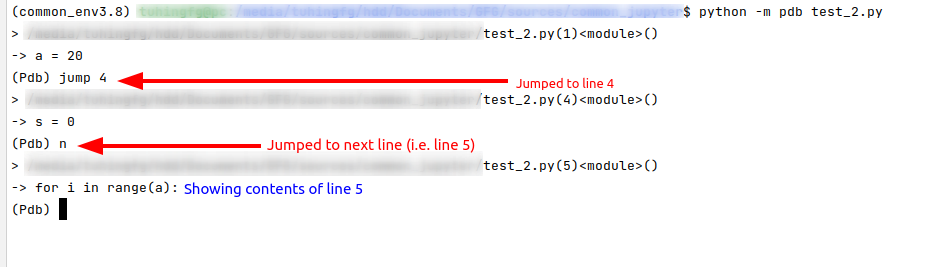Navigate in pdb prompt using commands

### Example 4: Post-mortem debugging using Python pdb module

Post-mortem debugging means entering debug mode after the program is finished with the execution process (failure has already occurred).  pdb supports post-mortem debugging through the pm() and post_mortem() functions. These functions look for active trace back and start the debugger at the line in the call stack where the exception occurred. In the output of the given example, you can notice pdb appear when an exception is encountered in the program.

## Python3

 `def` `multiply(a, b):``    ``answer ``=` `a ``*` `b``    ``return` `answer`  `x ``=` `input``(``"Enter first number : "``)``y ``=` `input``(``"Enter second number : "``)``result ``=` `multiply(x, y)``print``(result)`

Output :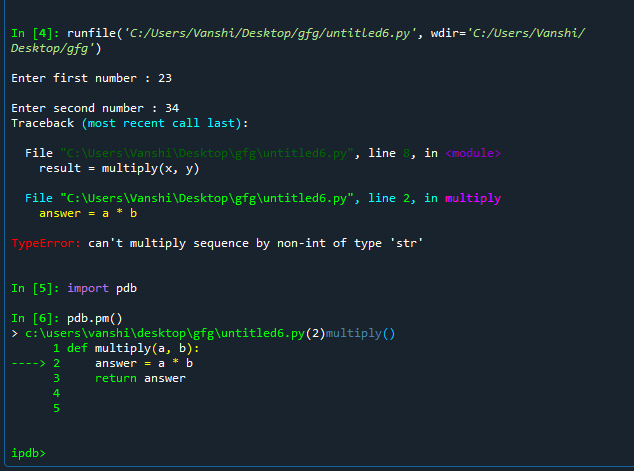### Checking variables on the Stack

All the variables including variables local to the function being executed in the program as well as global are maintained on the stack. We can use args(or use a) to print all the arguments of a function which is currently active. p command evaluates an expression given as an argument and prints the result.

Here, example 4 of this article is executed in debugging mode to show you how to check for variables :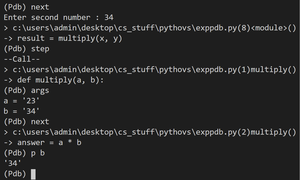checking variable values

### Python pdb Breakpoints

While working with large programs, we often want to add a number of breakpoints where we know errors might occur. To do this you just have to use the break command. When you insert a breakpoint, the debugger assigns a number to it starting from 1.  Use the break to display all the breakpoints in the program.

Syntax:

break filename: lineno, condition

Given below is the implementation to add breakpoints in a program used for example 4.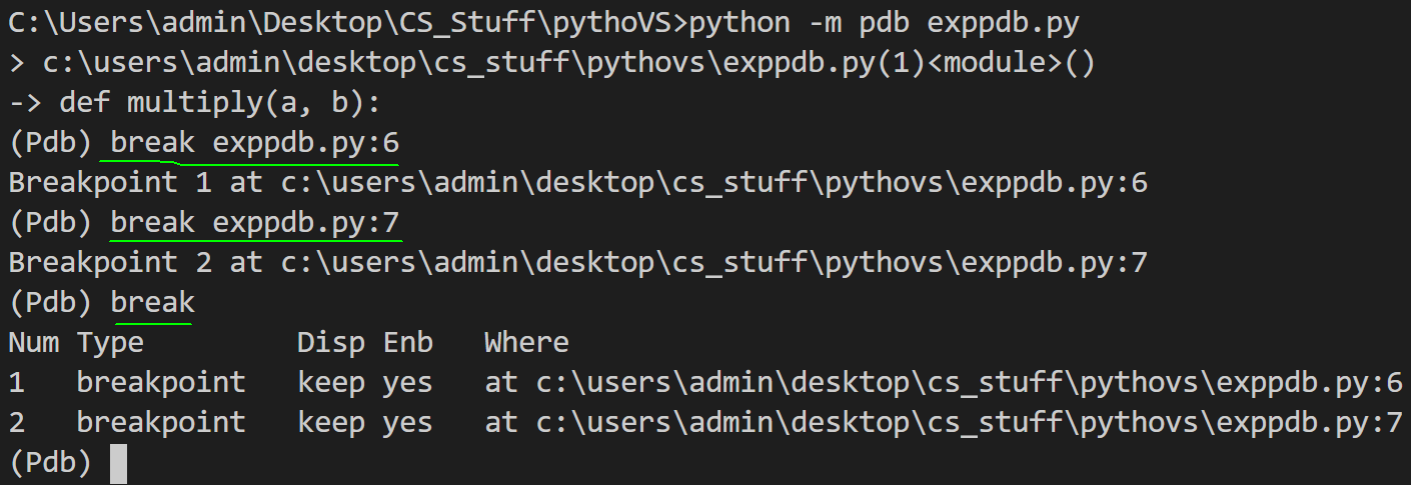### Managing Breakpoints

After adding breakpoints with the help of numbers assigned to them, we can manage the breakpoints using the enable and disable and remove command. disable tells the debugger not to stop when that breakpoint is reached, while enable turns on the disabled breakpoints.

Given below is the implementation to manage breakpoints using Example 4.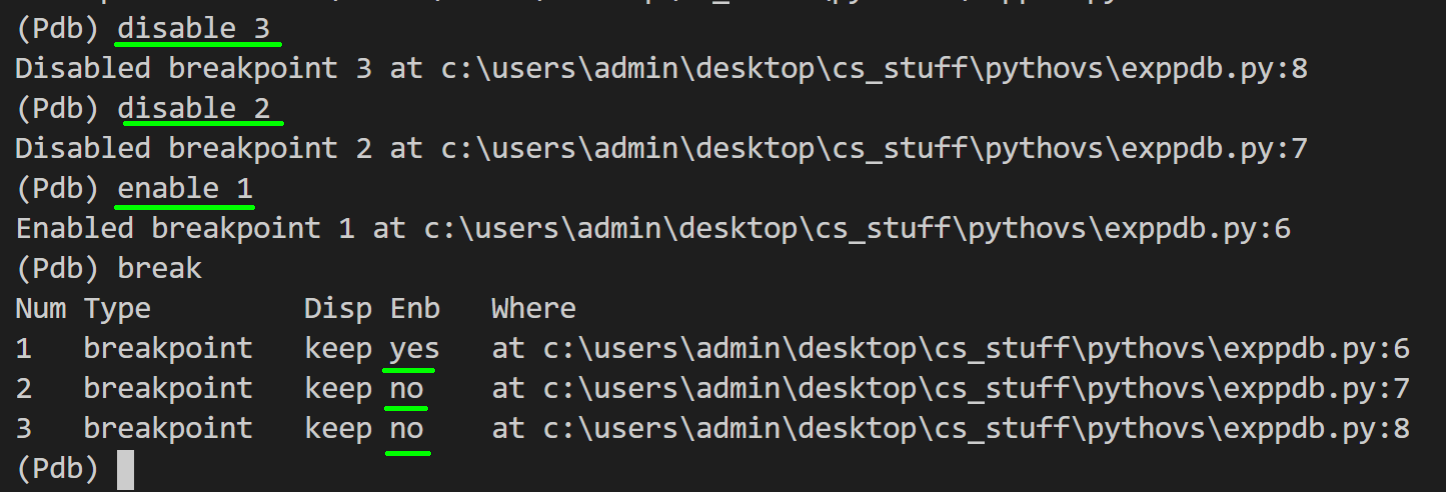Manage_breakpoints

My Personal Notes arrow_drop_up